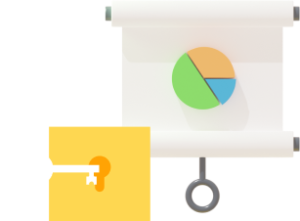Primary 6 Singapore math

# Primary 6 Algebra Made Easy

Primary 6 Algebra is a topic that can take awhile to get used to if you are a primary school student. However, if you are familiar with Singapore bar models, it might make things a bit easier. Written especially for Primary 6 (P6) kids and parents, this Algebra guide explains everything you need to know for Algebra in primary school before you get to do the more complex concepts in secondary school. Here’s what we’ll cover:

## What is Algebra in Primary 6 all about?

Algebra in Primary 6 is all about learning how to use letters in Mathematical sentences to represent unknown numbers. For example, let’s look at the letter n. n can represent any number. Its value may be 1, 5, 12, etc or take the form of simple fractions. Does that sound simple to you? Now, let’s move on to look at some Algebra rules.

### What are the rules of Algebra?

Just like everything else in Math, there are also rules that apply when we learn about Algebra in Primary 6. The simplest one is this. When we put a number and a letter together, for example, 2n, there is always an implicit multiplication sign in between them. This means that we can read 2n as 2 x n. In addition, when we see a letter on its own, we can assume that the number 1 is in front of the letter even though it is not written, so n is the same as 1 x n. Check out this Practicle Math video for an introduction to P6 Algebra:

### How are algebraic expressions formed?

Algebraic expressions can be made up of one or more of the 4 operations [Addition, Subtraction, Multiplication or Division] like the following:

#### The 3 Scenarios of Addition

I’m sure all of you are familiar with this 2 + 2. When we add 2 [a number] to 2 [another number], we get 4 [a number] as an answer. However, when we add a [a letter] to 2 [a number], we will leave our answer as a + 2 because we don’t know the value of a. The trick here is to think of the letter ‘a’ as a certain kind of box. Since we don’t know what is in the box, ‘a’ is left alone as ‘a’. Now what about a + a? If we have 1 certain kind of box and we add 1 more box of the same kind to it, we’ll get 2 of the same kind of boxes. That’s why a + a = 2a.

#### The 3 Scenarios of Subtraction

The same rules apply to subtraction.

#### Multiplication

Remember the multiplication rule in Algebra? 3 x n = 3n (*Refer to implicit rule when you see a number and a letter together)

#### Division

We usually express division in terms of a fraction.

### How do we simplify an algebraic expression?

Simplifying an algebraic expression makes it easier to work with. Let’s learn how to do that step by step. When the operations consist of only addition and/or subtraction, for example: 6d + 8 – 2d – 6 Step 1: Rearrange the terms such that all the terms with the letter are on one side and the numbers are on another: 6d – 2d + 8 – 6 Step 2: Simplify the expression by working it out: 4d + 2 When the operations consist of more than just addition and/or subtraction, for example: 2 x 8t + 5t – 10/2, apply the Order of Operations learnt in Primary 5.

1. Always start by solving the operation in the brackets.
2. Next, solve either division or multiplication from left to right
3. Then, solve addition or subtraction from left to right

Following those steps, we’ll simplify what is given as shown: 2 x 8t + 5t – 10/2 = 16t + 5t – 5 = 21t -5

### How do we do substitution in Algebra?

Replace the letter in the Math equation with the value of the unknown letter that is given. After that, follow the order of operation. You’ll be able to find the answer and solve the value of the entire algebraic expression. For example, if you are given that n = 7 and asked to find the value of of the algebraic expression 2n + 3, the way that we’ll work this out is this: 2n + 3 = (2 x 7) + 3 = 14 + 3 = 17

### Ready to try some Primary 6 Algebra practice questions?

Now that you have learnt everything you need to know about Algebra for Primary 6, try out some P6 Algebra practice questions! Check out these Primary 6 Algebra questions for practice and see how many you can solve!

### Experience Practicle free, no commitment no hidden cost no lock-in

Try our learning platform free with a 7 day trial and see if your child likes it.